# Simulation of Bouncing Ball

This example uses two bouncing ball Simulink® models to show different approaches to modeling hybrid dynamic systems with Zeno behavior. The example also provides the benefits of each approach.

Bouncing ball models are hybrid dynamic systems with the Zeno phenomenon. Zeno behavior is informally characterized by an infinite number of events occurring in a finite time interval for certain hybrid systems. As the ball loses energy, a large number of collisions with the ground start occurring in successively smaller intervals of time.

### Hybrid Dynamic Systems

A bouncing ball model is an example of a hybrid dynamic system. A hybrid dynamic system is a system that involves both continuous dynamics and discrete transitions where the system dynamics can change and the state values can jump. The continuous dynamics of a bouncing ball is given by these equations: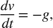whereis the acceleration due to gravity,is the position of the ball, andis the velocity. Therefore, the system has two continuous states: positionand velocity.

The hybrid system aspect of the model originates from the modeling of a collision of the ball with the ground. If one assumes a partially elastic collision with the ground, then the velocity before the collision,, and velocity after the collision,, can be related by the coefficient of restitution of the ball,, as follows:The bouncing ball therefore displays a jump in a continuous state (velocity) at the transition condition,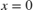. This figure shows a ball thrown up with a velocity of 0 m/s from a height of 25 m.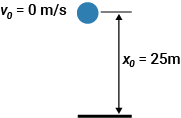### Use Two Integrator Blocks to Model Bouncing Ball

The sldemo_bounce_two_integrators model uses two Integrator blocks to model a bouncing ball. The Integrator block on the left is the velocity integrator modeling the first equation. The Integrator block on the right is the position integrator. Open the position integrator block dialog box and observe that the block has a lower limit of zero. This condition represents the constraint that the ball cannot go below the ground.

The state port of the position integrator and the corresponding comparison result is used to detect when the ball hits the ground and to reset both integrators. The state port of the velocity integrator is used for the calculation of.To observe the Zeno behavior of the system, go to the Solver pane of the Configuration Parameters dialog box. In Simulation time, set Stop time to 25.

• In Simulation time, set Stop time to 25.

• Expand Sovler details, In Zero-corssing options, set Algorithm to Nonadaptive.

Simulate the model.

As the ball hits the ground more frequently and loses energy, the simulation exceeds the Number of consecutive zero crossings default limit of 1000.

On the Configuration Parameters dialog box and go to Solver > Zero-crossing options. Set Algorithm to Adaptive. This algorithm introduces a sophisticated treatment for chattering behavior. You can now simulate the system beyond 20 seconds. Note, however, the chatter of the states between 21 seconds and 25 seconds and warning from Simulink about the strong chattering in the model around 20 seconds.

### Use Second-Order Integrator Block to Model Bouncing Ball

The sldemo_bounce model uses a single Second-Order Integrator block to model a bouncing ball. In this case, the second equation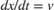is internal to the Second-Order Integrator block in this case. Open the Second-Order Integrator block dialog box and see thathas a lower limit of zero. On the Attributes tab, check Reinitialize dx/dt when x reaches saturation. This parameter allows you to reinitialize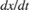(in the bouncing ball model) to a new value whenreaches its saturation limit. So, in the bouncing ball model, when the ball hits the ground, its velocity can be set to a different value, such as to the velocity after the impact. Note the loop for calculating the velocity after a collision with the ground. To capture the velocityof the ball just before the collision, theoutput port of the Second-Order Integrator block and a Memory block are used.is then used to calculate the rebound velocity.In the Configuration Parameters dialog box, go to the Solver pane.

• In Simulation time, set Stop time to 25.

• Expand Solver details. In Zero-crossing options, set Algorithm to Nonadaptive .

Simulate the model.

Note that the simulation encounters no problems. You can simulate the model without experiencing excessive chatter after 20 seconds and without setting Algorithm to Adaptive.

### Compare Approaches to Modeling Bouncing Ball

You can analytically calculate the exact timewhen the ball settles down to the ground with zero velocity by summing the time required for each bounce. This time is the sum of an infinite geometric series given by:whereand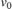are initial conditions for position and velocity, respectively. The velocity and the position of the ball must be identically zero for. The figure shows results from both simulations near. The vertical red line in the plot isfor the given model parameters. Forand far away from, both models produce accurate and identical results. Only a magenta line from the second model is visible in the plot. However, the simulation results from the first model are inexact after. The plot continues to display excessive chattering behavior for. In contrast, the model that uses the Second-Order Integrator block settles to exactly zero for.The model that uses the Second-Order Integrator block has superior numerical characteristics compared to the first model because the second differential equationis internal to the Second-Order Integrator block. The block algorithms can leverage this relationship between the two states and use heuristics to clamp down chattering behavior for certain conditions. These heuristics become active when the two states are no longer mutually consistent due to integration errors and chattering behavior. You can thus use physical knowledge of the system to prevent simulations getting stuck in a Zeno state for certain classes of Zeno models.